# armafevd

Generate or plot ARMA model forecast error variance decomposition (FEVD)

## Syntax

``armafevd(ar0,ma0)``
``armafevd(ar0,ma0,Name,Value)``
``Y = armafevd(___)``
``armafevd(ax,___)``
``[Y,h] = armafevd(___)``

## Description

The `armafevd` function returns or plots the forecast error variance decomposition of the variables in a univariate or vector (multivariate) autoregressive moving average (ARMA or VARMA) model specified by arrays of coefficients or lag operator polynomials.

Alternatively, you can return an FEVD from a fully specified (for example, estimated) model object by using a function in this table.

The FEVD provides information about the relative importance of each innovation in affecting the forecast error variance of all variables in the system. In contrast, the impulse response function (IRF) traces the effects of an innovation shock to one variable on the response of all variables in the system. To estimate IRFs of univariate or multivariate ARMA models, see `armairf`.

example

````armafevd(ar0,ma0)` plots, in separate figures, the FEVD of the `numVars` time series variables that compose an ARMA(p,q) model, with autoregressive (AR) and moving average (MA) coefficients `ar0` and `ma0`, respectively. Each figure corresponds to a variable and contains `numVars` line plots. The line plots are the FEVDs of that variable, over the forecast horizon, resulting from a one-standard-deviation innovation shock applied to all variables in the system at time 0.The `armafevd` function: Accepts vectors or cell vectors of matrices in difference-equation notationAccepts `LagOp` lag operator polynomials corresponding to the AR and MA polynomials in lag operator notationAccommodates time series models that are univariate or multivariate, stationary or integrated, structural or in reduced form, and invertible or noninvertibleAssumes that the model constant c is 0 ```

example

``armafevd(ar0,ma0,Name,Value)` plots the `numVars` FEVDs with additional options specified by one or more name-value pair arguments. For example, `'NumObs',10,'Method',"generalized"` specifies a 10-period forecast horizon and the estimation of the generalized FEVD.`

example

``Y = armafevd(___)` returns the `numVars` FEVDs using any of the input argument combinations in the previous syntaxes.`
````armafevd(ax,___)` plots to the axes specified in `ax` instead of the axes in new figures. The option `ax` can precede any of the input argument combinations in the previous syntaxes.```
````[Y,h] = armafevd(___)` additionally returns handles to plotted graphics objects. Use elements of `h` to modify properties of the returned plots.```

## Examples

collapse all

Plot the FEVD of the univariate ARMA(2,1) model

`${y}_{t}=0.3{y}_{t-1}-0.1{y}_{t-2}+{\epsilon }_{t}+0.05{\epsilon }_{t-1}.$`

Create vectors for the autoregressive and moving average coefficients as you encounter them in the model, which is expressed in difference-equation notation.

```AR0 = [0.3 -0.1]; MA0 = 0.05;```

Plot the orthogonalized FEVD of ${y}_{t}$.

`armafevd(AR0,MA0);`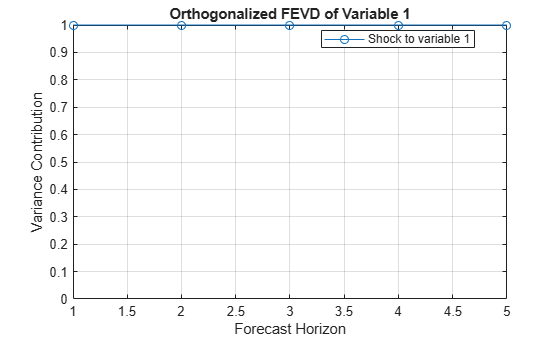Because ${y}_{t}$ is univariate, the FEVD is trivial.

Plot the FEVD of the VARMA(3,1) model

`$\begin{array}{c}{y}_{t}=\left[\begin{array}{ccc}-0.5& 0.2& 0.1\\ 0.3& 0.1& -0.1\\ -0.4& 0.2& 0.05\end{array}\right]{y}_{t-1}+\left[\begin{array}{ccc}-0.05& 0.02& 0.01\\ 0.1& 0.01& 0.001\\ -0.04& 0.02& 0.005\end{array}\right]{y}_{t-3}+{\epsilon }_{t}+\left[\begin{array}{ccc}-0.02& 0.03& 0.3\\ 0.003& 0.001& 0.01\\ 0.3& 0.01& 0.01\end{array}\right]{\epsilon }_{t-1}\end{array}$`

where ${y}_{t}={\left[{y}_{1t}\phantom{\rule{0.2777777777777778em}{0ex}}\phantom{\rule{0.2777777777777778em}{0ex}}\phantom{\rule{0.2777777777777778em}{0ex}}{y}_{2t}\phantom{\rule{0.2777777777777778em}{0ex}}\phantom{\rule{0.2777777777777778em}{0ex}}\phantom{\rule{0.2777777777777778em}{0ex}}{y}_{3t}\right]}^{\prime }$ and ${\epsilon }_{t}={\left[{\epsilon }_{1t}\phantom{\rule{0.2777777777777778em}{0ex}}\phantom{\rule{0.2777777777777778em}{0ex}}\phantom{\rule{0.2777777777777778em}{0ex}}{\epsilon }_{2t}\phantom{\rule{0.2777777777777778em}{0ex}}\phantom{\rule{0.2777777777777778em}{0ex}}\phantom{\rule{0.2777777777777778em}{0ex}}{\epsilon }_{3t}\right]}^{\prime }$.

The VARMA model is in difference-equation notation because the current response is isolated from all other terms in the equation.

Create a cell vector containing the VAR matrix coefficients. The position of the coefficient matrix in the cell vector determines its lag. Therefore, specify a 3-by-3 matrix of zeros as the second element of the vector.

```var0 = {[-0.5 0.2 0.1; 0.3 0.1 -0.1; -0.4 0.2 0.05],... zeros(3),... [-0.05 0.02 0.01; 0.1 0.01 0.001; -0.04 0.02 0.005]};```

Create a cell vector containing the VMA matrix coefficients.

`vma0 = {[-0.02 0.03 0.3; 0.003 0.001 0.01; 0.3 0.01 0.01]};`

Plot the orthogonalized FEVDs of the VARMA model.

`armafevd(var0,vma0);`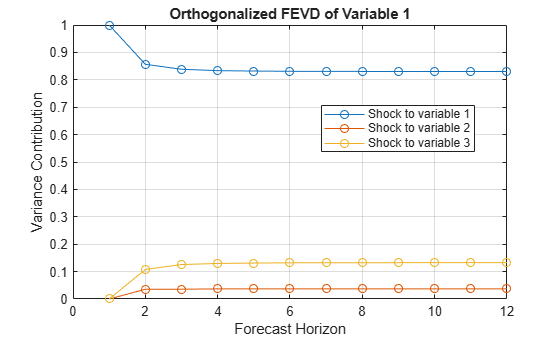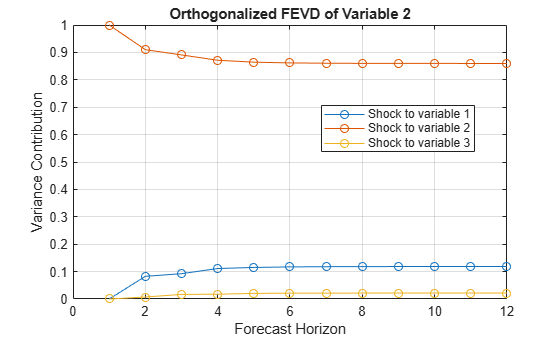`armafevd` returns three figures. Figure k contains the generalized FEVD of variable k to a shock applied to all other variables at time 0.

• You can attribute most of the forecast error variance of variable 1 to a shock to variable 1. A shock to variable 2 does not contribute much to the forecast error variance of variable 1.

• You can attribute most of the forecast error variance of variable 2 to a shock to variable 2. A shock to variable 3 does not contribute much to the forecast error variance of variable 2.

• You can attribute most of the forecast error variance of variable 3 to a shock to variable 3. A shock to variable 2 does not contribute much to the forecast error variance of variable 3.

Plot the entire FEVD of the structural VARMA(8,4) model

`$\begin{array}{l}\left\{\left[\begin{array}{ccc}1& 0.2& -0.1\\ 0.03& 1& -0.15\\ 0.9& -0.25& 1\end{array}\right]-\left[\begin{array}{ccc}-0.5& 0.2& 0.1\\ 0.3& 0.1& -0.1\\ -0.4& 0.2& 0.05\end{array}\right]{L}^{4}-\left[\begin{array}{ccc}-0.05& 0.02& 0.01\\ 0.1& 0.01& 0.001\\ -0.04& 0.02& 0.005\end{array}\right]{L}^{8}\right\}{y}_{t}=\\ \left\{\left[\begin{array}{ccc}1& 0& 0\\ 0& 1& 0\\ 0& 0& 1\end{array}\right]+\left[\begin{array}{ccc}-0.02& 0.03& 0.3\\ 0.003& 0.001& 0.01\\ 0.3& 0.01& 0.01\end{array}\right]{L}^{4}\right\}{\epsilon }_{t}\end{array}$`

where ${y}_{t}={\left[{y}_{1t}\phantom{\rule{0.2777777777777778em}{0ex}}\phantom{\rule{0.2777777777777778em}{0ex}}\phantom{\rule{0.2777777777777778em}{0ex}}{y}_{2t}\phantom{\rule{0.2777777777777778em}{0ex}}\phantom{\rule{0.2777777777777778em}{0ex}}\phantom{\rule{0.2777777777777778em}{0ex}}{y}_{3t}\right]}^{\prime }$ and ${\epsilon }_{t}={\left[{\epsilon }_{1t}\phantom{\rule{0.2777777777777778em}{0ex}}\phantom{\rule{0.2777777777777778em}{0ex}}\phantom{\rule{0.2777777777777778em}{0ex}}{\epsilon }_{2t}\phantom{\rule{0.2777777777777778em}{0ex}}\phantom{\rule{0.2777777777777778em}{0ex}}\phantom{\rule{0.2777777777777778em}{0ex}}{\epsilon }_{3t}\right]}^{\prime }$.

The VARMA model is in lag operator notation because the response and innovation vectors are on opposite sides of the equation.

Create a cell vector containing the VAR matrix coefficients. Because this model is a structural model in lag operator notation, start with the coefficient of ${y}_{t}$ and enter the rest in order by lag. Construct a vector that indicates the degree of the lag term for the corresponding coefficients (the structural-coefficient lag is 0).

```var0 = {[1 0.2 -0.1; 0.03 1 -0.15; 0.9 -0.25 1],... -[-0.5 0.2 0.1; 0.3 0.1 -0.1; -0.4 0.2 0.05],... -[-0.05 0.02 0.01; 0.1 0.01 0.001; -0.04 0.02 0.005]}; var0Lags = [0 4 8];```

Create a cell vector containing the VMA matrix coefficients. Because this model is in lag operator notation, start with the coefficient of ${\epsilon }_{t}$ and enter the rest in order by lag. Construct a vector that indicates the degree of the lag term for the corresponding coefficients.

```vma0 = {eye(3),... [-0.02 0.03 0.3; 0.003 0.001 0.01; 0.3 0.01 0.01]}; vma0Lags = [0 4];```

Construct separate lag operator polynomials that describe the VAR and VMA components of the VARMA model.

```VARLag = LagOp(var0,'Lags',var0Lags); VMALag = LagOp(vma0,'Lags',vma0Lags);```

Plot the generalized FEVDs of the VARMA model.

`armafevd(VARLag,VMALag,'Method','generalized');`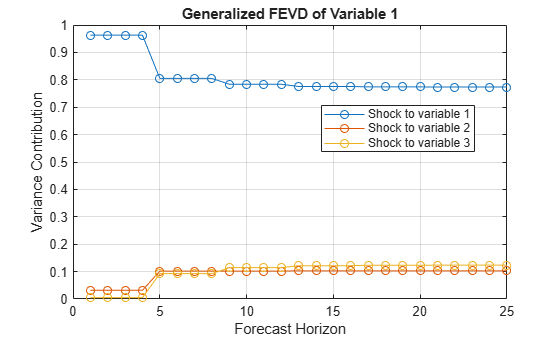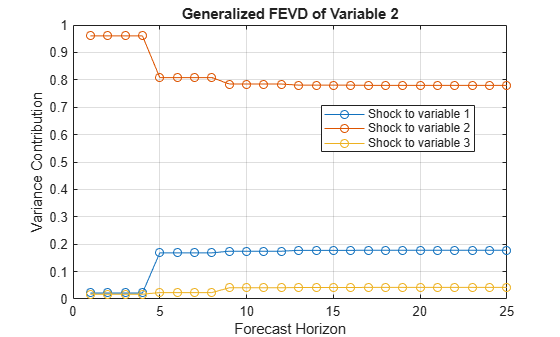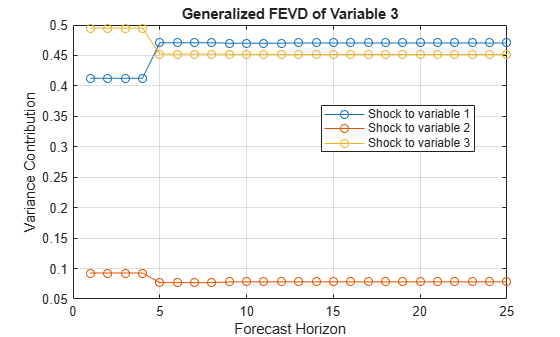`armafevd` returns three figures. Figure k contains the generalized FEVD of variable k to a shock applied to all other variables at time 0.

• You can attribute most of the forecast error variance of variable 1 to a shock to variable 1. Shocks to variables 2 and 3 contribute similarly to the forecast error variance of variable 1.

• You can attribute most of the forecast error variance of variable 2 to a shock to variable 2. A shock to variable 3 does not contribute much to the forecast error variance of variable 2.

• You can attribute most of the forecast error variance of variable 3 to shocks to variables 1 and 3, each contributing similar amounts. A shock to variable 2 does not contribute much to the forecast error variance of variable 3.

Compute the generalized FEVDs of the two-dimensional VAR(3) model

`${y}_{t}=\left[\begin{array}{cc}1& -0.2\\ -0.1& 0.3\end{array}\right]{y}_{t-1}-\left[\begin{array}{cc}0.75& -0.1\\ -0.05& 0.15\end{array}\right]{y}_{t-2}+\left[\begin{array}{cc}0.55& -0.02\\ -0.01& 0.03\end{array}\right]{y}_{t-3}+{\epsilon }_{t}.$`

In the equation, ${y}_{t}=\left[{y}_{1,t}\phantom{\rule{0.2777777777777778em}{0ex}}\phantom{\rule{0.2777777777777778em}{0ex}}\phantom{\rule{0.2777777777777778em}{0ex}}{y}_{2,t}{\right]}^{\prime }$, ${\epsilon }_{t}=\left[{\epsilon }_{1,t}\phantom{\rule{0.2777777777777778em}{0ex}}\phantom{\rule{0.2777777777777778em}{0ex}}\phantom{\rule{0.2777777777777778em}{0ex}}{\epsilon }_{2,t}{\right]}^{\prime }$, and, for all t, ${\epsilon }_{t}$ is Gaussian with mean zero and covariance matrix

`$\Sigma =\left[\begin{array}{cc}0.5& -0.1\\ -0.1& 0.25\end{array}\right].$`

Create a cell vector of matrices for the autoregressive coefficients as you encounter them in the model as expressed in difference-equation notation. Specify the innovation covariance matrix.

```AR1 = [1 -0.2; -0.1 0.3]; AR2 = -[0.75 -0.1; -0.05 0.15]; AR3 = [0.55 -0.02; -0.01 0.03]; ar0 = {AR1 AR2 AR3}; InnovCov = [0.5 -0.1; -0.1 0.25];```

Compute the generalized FEVDs of ${y}_{t}$. Because no MA terms exist, specify an empty array (`[]`) for the second input argument.

```Y = armafevd(ar0,[],'Method','generalized','InnovCov',InnovCov); size(Y)```
```ans = 1×3 31 2 2 ```
`Y(10,1,2)`
```ans = 0.1302 ```

`Y` is a 31-by-2-by-2 array of FEVDs. Rows correspond to times 1 through 31 in the forecast horizon, columns correspond to the variables that `armafevd` shocks at time 0, and pages correspond to the FEVD of the variables in the system. For example, the contribution to the forecast error variance of variable 2 at time 10 in the forecast horizon, attributable to a shock to variable 1, is `Y(10,1,2)` = 0.1302.

`armafevd` satisfies the stopping criterion after 31 periods. You can specify to stop sooner using the `'NumObs'` name-value pair argument. This practice is beneficial when the system has many variables.

Compute and display the generalized FEVDs for the first 10 periods.

```Y10 = armafevd(ar0,[],'Method','generalized','InnovCov',InnovCov,... 'NumObs',10)```
```Y10 = Y10(:,:,1) = 1.0000 0.0800 0.9912 0.1238 0.9863 0.1343 0.9863 0.1341 0.9873 0.1294 0.9874 0.1313 0.9864 0.1342 0.9864 0.1343 0.9866 0.1336 0.9867 0.1336 Y10(:,:,2) = 0.0800 1.0000 0.1157 0.9838 0.1235 0.9737 0.1236 0.9737 0.1237 0.9736 0.1264 0.9709 0.1296 0.9679 0.1298 0.9677 0.1298 0.9677 0.1302 0.9673 ```

`Y10` is a 10-by-2-by-2 array of FEVDs. Rows correspond to times 1 through 10 in the forecast horizon. In all FEVDs, the contributions appear to stabilize before 10 periods elapse.

For each variable (page), compute the row sums.

`sum(Y10,2)`
```ans = ans(:,:,1) = 1.0800 1.1150 1.1206 1.1204 1.1167 1.1187 1.1206 1.1207 1.1202 1.1203 ans(:,:,2) = 1.0800 1.0995 1.0972 1.0973 1.0973 1.0973 1.0975 1.0975 1.0975 1.0975 ```

For generalized FEVDs, forecast error variance contributions at each period in the forecast horizon do not necessarily sum to one. This characteristic is in contrast to orthogonalized FEVDs, in which all rows sum to one.

## Input Arguments

collapse all

Autoregressive coefficients of the ARMA(p,q) model, specified as a numeric vector, cell vector of square numeric matrices, or `LagOp` lag operator polynomial object. If `ar0` is a vector (numeric or cell), then the coefficient of yt is the identity (`eye(numVars)`).

For an MA model, specify an empty array or cell (`[]` or `{}`).

• For univariate time series models, `ar0` is a numeric vector, cell vector of scalars, or one-dimensional `LagOp` lag operator polynomial. For vectors, `ar0` has length p, and the elements correspond to lagged responses that compose the AR polynomial in difference-equation notation. In other words, `ar0(j)` or `ar0{j}` is the coefficient of yt-j, j = 1,…,p. Variance decompositions of univariate models are trivial; see `Y`.

• For `numVars`-dimensional time series models, `ar0` is a cell vector of `numVars`-by-`numVars` numeric matrices or a `numVars`-dimensional `LagOp` lag operator polynomial. For cell vectors:

• `ar0` has length p.

• `ar0` and `ma0` each must contain `numVars`-by-`numVars` matrices. For each matrix, row k and column k correspond to variable k in the system k = 1,…,`numVars`.

• The elements of `ar0` correspond to the lagged responses that compose the AR polynomial in difference equation notation. In other words, `ar0{j}` is the coefficient matrix of vector yt-j, `j` = 1,…,p. For all AR coefficient matrices, row k contains the AR coefficients in the equation of the variable ykt, and column k contains the coefficients of variable ykt within the equations. The row and column order of all autoregressive and moving average coefficients must be consistent.

• For `LagOp` lag operator polynomials:

• Coefficients in the `Coefficients` property correspond to the lags of yt in the `Lags` property.

• Specify a model in reduced form by supplying the identity for the first coefficient (`eye(numVars)`).

• `armafevd` composes the model using lag operator notation. In other words, when you work from a model in difference-equation notation, negate the AR coefficients of the lagged responses to construct the lag operator polynomial equivalent.

For example, consider ${y}_{t}=0.5{y}_{t-1}-0.8{y}_{t-2}+{\epsilon }_{t}-0.6{\epsilon }_{t-1}+0.08{\epsilon }_{t-2}$. The model is in difference-equation form. To compute the FEVD, enter the following at the command line.

`y = armafevd([0.5 -0.8], [-0.6 0.08]);`

The ARMA model written in lag operator notation is $\left(1-0.5L+0.8{L}^{2}\right){y}_{t}=\left(1-0.6L+0.08{L}^{2}\right){\epsilon }_{t}.$ The AR coefficients of the lagged responses are negated compared to the corresponding coefficients in difference-equation format. To obtain the same result using lag operator notation, enter the following at the command line.

```ar0 = LagOp({1 -0.5 0.8}); ma0 = LagOp({1 -0.6 0.08}); y = armafevd(ar0, ma0);```

Moving average coefficients of the ARMA(p,q) model, specified as a numeric vector, cell vector of square numeric matrices, or `LagOp` lag operator polynomial object. If `ma0` is a vector (numeric or cell), then the coefficient of εt is the identity (`eye(numVars)`).

For an AR model, specify an empty array or cell (`[]` or `{}`).

• For univariate time series models, `ma0` is a numeric vector, cell vector of scalars, or one-dimensional `LagOp` lag operator polynomial. For vectors, `ma0` has length q, and the elements correspond to lagged innovations that compose the AR polynomial in difference-equation notation. In other words, `ma0(j)` or `ma0{j}` is the coefficient of εt-j, j = 1,…,q. Variance decompositions of univariate models are trivial; see `Y`.

• For `numVars`-dimensional time series models, `ma0` is a cell vector of numeric `numVars`-by-`numVars` numeric matrices or a `numVars`-dimensional `LagOp` lag operator polynomial. For cell vectors:

• `ma0` has length q.

• `ar0` and `ma0` each must contain `numVars`-by-`numVars` matrices. For each matrix, row k and column k correspond to variable k in the system k = 1,…,`numVars`.

• The elements of `ma0` correspond to the lagged responses that compose the MA polynomial in difference-equation notation. In other words, `ma0{j}` is the coefficient matrix of εt-j, `j` = 1,…,q. For all MA coefficient matrices, row k contains the MA coefficients in the equation of the variable εkt, and column k contains the coefficients of εkt within the equations. The row and column order of all autoregressive and moving average coefficient matrices must be consistent.

• For `LagOp` lag operator polynomials, coefficients in the `Coefficients` property correspond to the lags of εt in the `Lags` property.

To specify a model in reduced form, supply the identity (`eye(numVars)`) for the coefficient that corresponds to lag 0.

Axes on which to plot the FEVD of each variable, specified as a vector of `Axes` objects with length equal to `numVars`.

By default, `armafevd` plots variance decompositions on axes in separate figures.

### Name-Value Arguments

Specify optional comma-separated pairs of `Name,Value` arguments. `Name` is the argument name and `Value` is the corresponding value. `Name` must appear inside quotes. You can specify several name and value pair arguments in any order as `Name1,Value1,...,NameN,ValueN`.

Example: `'Method',"generalized",'NumObs',10` specifies to compute the generalized FEVD of each variable for 10 periods.

Covariance matrix of the ARMA(p,q) model innovations εt, specified as the comma-separated pair consisting of `'InnovCov'` and a numeric scalar or a `numVars`-by-`numVars` numeric matrix. `InnovCov` must be a positive scalar or a positive definite matrix.

The default value is `eye(numVars)`.

Example: `'InnovCov',0.2`

Data Types: `double`

Forecast horizon, or the number of periods for which `armafevd` computes the FEVD, specified as the comma-separated pair consisting of `'NumObs'` and a positive integer. In other words, `NumObs` specifies the number of observations to include in the FEVD (the number of rows in `Y`).

By default, `armafevd` determines `NumObs` by the stopping criteria of `mldivide`.

Example: `'NumObs',10`

Data Types: `double`

FEVD computation method, specified as the comma-separated pair consisting of `'Method'` and a value in this table.

ValueDescription
`"orthogonalized"`Compute variance decompositions using orthogonalized, one-standard-deviation innovation shocks. `armafevd` uses the Cholesky factorization of `InnovCov` for orthogonalization.
`"generalized"`Compute variance decompositions using one-standard-deviation innovation shocks.

Example: `'Method',"generalized"`

Data Types: `char` | `string`

## Output Arguments

collapse all

FEVD of each variable, returned as a column vector of ones or a numeric array.

`Y(t,j,k)` is the contribution to the variance decomposition of variable `k` attributable to an innovation shock to variable `j` at time `t`, for `t` = 1,2,…,`numObs`, `j` = 1,2,...,`numVars`, and `k` = 1,2,...,`numVars`. The columns and pages of `Y` correspond to the variable order in `ar0` and `ma0`.

For univariate models, `Y` is `ones(numObs,1)` because the variance decomposition is one for each period in the forecast horizon.

Handles to plotted graphics objects, returned as a `numVars`-by-`numVars` matrix of graphics objects. `h(j,k)` corresponds to the FEVD of `k` attributable to an innovation shock to variable `j` at time 0.

`h` contains unique plot identifiers, which you can use to query or modify properties of the plot.

collapse all

### Difference-Equation Notation

A linear time series model written in difference-equation notation positions the present value of the response and its structural coefficient on the left side of the equation. The right side of the equation contains the sum of the lagged responses, present innovation, and lagged innovations with corresponding coefficients.

In other words, a linear time series written in difference-equation notation is

`${\Phi }_{0}{y}_{t}=c+{\Phi }_{1}{y}_{t-1}+...+{\Phi }_{p}{y}_{t-p}+{\Theta }_{0}{\epsilon }_{t}+{\Theta }_{1}{\epsilon }_{t-1}+...+{\Theta }_{q}{\epsilon }_{t-q},$`

where

• yt is a `numVars`-dimensional vector representing the responses of `numVars` variables at time t, for all t and for `numVars` ≥ 1.

• εt is a `numVars`-dimensional vector representing the innovations at time t.

• Φj is the `numVars`-by-`numVars` matrix of AR coefficients of the response yt-j, for j = 0,...,p.

• Θk is the `numVars`-by-`numVars` matrix of MA coefficients of the innovation εt-k., k = 0,...,q.

• c is the n-dimensional model constant.

• Φ0 = Θ0 = InumVars, which is the `numVars`-dimensional identity matrix, for models in reduced form.

### Forecast Error Variance Decomposition

The forecast error variance decomposition (FEVD) of a multivariate, dynamic system shows the relative importance of a shock to each innovation in affecting the forecast error variance of all variables in the system.

Suppose yt is the ARMA(p,q) model containing `numVars` response variables

`$\Phi \left(L\right){y}_{t}=\Theta \left(L\right){\epsilon }_{t}.$`

• Φ(L) is the lag operator polynomial of the autoregressive coefficients, in other words, $\Phi \left(L\right)={\Phi }_{0}-{\Phi }_{1}L-{\Phi }_{2}{L}^{2}-...-{\Phi }_{p}{L}^{p}.$

• Θ(L) is the lag operator polynomial of the moving average coefficients, in other words, $\Theta \left(L\right)={\Theta }_{0}+{\Theta }_{1}L+{\Theta }_{2}{L}^{2}+...+{\Theta }_{q}{L}^{q}.$

• εt is the vector of `numVars`-D series of innovations . Assume that the innovations have zero mean and the constant, positive-definite covariance matrix Σ for all t.

The infinite-lag MA representation of yt is

`${y}_{t}={\Phi }^{-1}\left(L\right)\Theta \left(L\right){\epsilon }_{t}=\Omega \left(L\right){\epsilon }_{t}.$`

The general form of the FEVD of ykt (variable k) m periods into the future, attributable to a one-standard-deviation innovation shock to yjt, is

`${\gamma }_{mjk}=\frac{\sum _{t=0}^{m-1}{\left({e}_{k}{}^{\prime }{C}_{t}{e}_{j}\right)}^{2}}{\sum _{t=0}^{m-1}{e}_{k}^{\prime }{\Omega }_{t}\Sigma {\Omega }_{t}^{\prime }{e}_{k}}.$`
• ej is a selection vector of length `numVars` containing a one in element j and zeros elsewhere.

• For orthogonalized FEVDs, ${C}_{m}={\Omega }_{m}P,$ where P is the lower triangular factor in the Cholesky factorization of Σ.

• For generalized FEVDs, ${C}_{m}={\sigma }_{j}^{-1}{\Omega }_{m}\Sigma ,$ where σj is the standard deviation of innovation j.

• The numerator is the contribution of an innovation shock to variable j to the forecast error variance of the m-step ahead forecast of variable k. The denominator is the mean squared error (MSE) of the m-step ahead forecast of variable k .

### Lag Operator Notation

A time series model written in lag operator notation positions a p-degree lag operator polynomial on the present response on the left side of the equation. The right side of the equation contains the model constant and a q-degree lag operator polynomial on the present innovation.

In other words, a linear time series model written in lag operator notation is

`$\Phi \left(L\right){y}_{t}=c+\Theta \left(L\right){\epsilon }_{t},$`

where

• yt is a `numVars`-dimensional vector representing the responses of `numVars` variables at time t, for all t and for `numVars` ≥ 1.

• $\Phi \left(L\right)={\Phi }_{0}-{\Phi }_{1}L-{\Phi }_{2}{L}^{2}-...-{\Phi }_{p}{L}^{p}$, which is the autoregressive, lag operator polynomial.

• L is the back-shift operator, in other words, ${L}^{j}{y}_{t}={y}_{t-j}$.

• Φj is the `numVars`-by-`numVars` matrix of AR coefficients of the response yt-j, for j = 0,...,p.

• εt is a `numVars`-dimensional vector representing the innovations at time t.

• $\Theta \left(L\right)={\Theta }_{0}+{\Theta }_{1}L+{\Theta }_{2}{L}^{2}+...+{\Theta }_{q}{L}^{q}$, which is the moving average, lag operator polynomial.

• Θk is the `numVars`-by-`numVars` matrix of MA coefficients of the innovation εt-k., k = 0,...,q.

• c is the `numVars`-dimensional model constant.

• Φ0 = Θ0 = I`numVars`, which is the `numVars`-dimensional identity matrix, for models in reduced form.

When comparing lag operator notation to difference-equation notation, the signs of the lagged AR coefficients appear negated relative to the corresponding terms in difference-equation notation. The signs of the moving average coefficients are the same and appear on the same side.

For more details on lag operator notation, see Lag Operator Notation.

## Tips

• To accommodate structural ARMA(p,q) models, supply `LagOp` lag operator polynomials for the input arguments `ar0` and `ma0`. To specify a structural coefficient when you call `LagOp`, set the corresponding lag to 0 by using the `'Lags'` name-value pair argument.

• For orthogonalized multivariate FEVDs, arrange the variables according to Wold causal ordering :

• The first variable (corresponding to the first row and column of both `ar0` and `ma0`) is most likely to have an immediate impact (t = 0) on all other variables.

• The second variable (corresponding to the second row and column of both `ar0` and `ma0`) is most likely to have an immediate impact on the remaining variables, but not the first variable.

• In general, variable j (corresponding to row j and column j of both `ar0` and `ma0`) is the most likely to have an immediate impact on the last `numVars`j variables, but not the previous j – 1 variables.

## Algorithms

• `armafevd` plots FEVDs only when it returns no output arguments or `h`.

• If `Method` is `"orthogonalized"`, then `armafevd` orthogonalizes the innovation shocks by applying the Cholesky factorization of the innovations covariance matrix `InnovCov`. The covariance of the orthogonalized innovation shocks is the identity matrix, and the FEVD of each variable sums to one, that is, the sum along any row of `Y` is one. Therefore, the orthogonalized FEVD represents the proportion of forecast error variance attributable to various shocks in the system. However, the orthogonalized FEVD generally depends on the order of the variables.

If `Method` is `"generalized"`, then:

• The resulting FEVD is invariant to the order of the variables.

• The resulting FEVD is not based on an orthogonal transformation.

• The resulting FEVD of a variable sums to one only when `InnovCov` is diagonal .

Therefore, the generalized FEVD represents the contribution to the forecast error variance of equation-wise shocks to the variables in the system.

• If `InnovCov` is a diagonal matrix, then the resulting generalized and orthogonalized FEVDs are identical. Otherwise, the resulting generalized and orthogonalized FEVDs are identical only when the first variable shocks all variables (in other words, all else being the same, both methods yield the same value of `Y(:,1,:)`).

 Hamilton, James D. Time Series Analysis. Princeton, NJ: Princeton University Press, 1994.

 Lütkepohl, H. "Asymptotic Distributions of Impulse Response Functions and Forecast Error Variance Decompositions of Vector Autoregressive Models." Review of Economics and Statistics. Vol. 72, 1990, pp. 116–125.

 Lütkepohl, Helmut. New Introduction to Multiple Time Series Analysis. New York, NY: Springer-Verlag, 2007.

 Pesaran, H. H., and Y. Shin. "Generalized Impulse Response Analysis in Linear Multivariate Models." Economic Letters. Vol. 58, 1998, pp. 17–29.